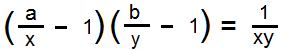Find the number of integer solutions for x and y to the equation :Input Specifications
First line contains the number of test cases. For each test case there is one line containing two integers a and b.

Output Specifications
Output single integer for every test case which should be the the number of integer solutions to the given equation.

Constraints
-1000 <= a,b <= 1000
No. of test cases <= 100

Sample Input``` 3 6 7 1 3 1 -1```

Sample Output``` 2 1 0```

Sample Explanation
Sample Case 1 : (5,6) and (7,8)
Sample Case 2 : (2,4)
Sample Case 3 : No integer solution

Languages: AWK,Bash,Brain,C,C++,Java,C#,JavaScript,Pascal,Perl,PHP,Python,Python3,Ruby,Text

Time Limit: 1 Second(s)
Score: 100 Point(s)
Input File Limit: 50000 Bytes

Mode Judge

RankNameScore
1xyz0
2Ams0
3TIP0
4team420
5xyzz0
6asdasdasd0
7abcd0
8khankhan0
9Gabriel0
10gigel0